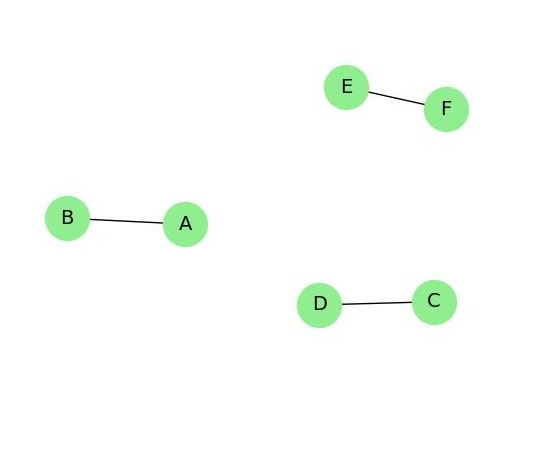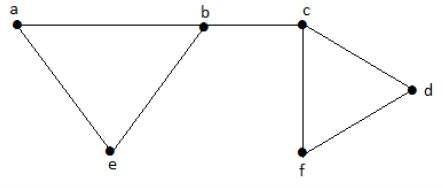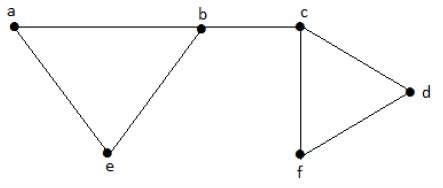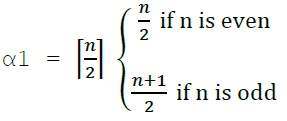# Independent Line Set

Independent sets are represented in sets, in which

• there should not be any edges adjacent to each other. There should not be any common vertex between any two edges.

• there should not be any vertices adjacent to each other. There should not be any common edge between any two vertices.

## Independent Line Set

Let 'G' = (V, E) be a graph. A subset L of E is called an independent line set of 'G' if no two edges in L are adjacent. Such a set is called an independent line set.

## ExampleLet us consider the following subsets −

L1 = {a,b}
L2 = {a,b} {c,e}
L3 = {a,d} {b,c}

In this example, the subsets L2 and L3 are clearly not the adjacent edges in the given graph. They are independent line sets. However L1 is not an independent line set, as for making an independent line set, there should be at least two edges.

## Maximal Independent Line Set

An independent line set is said to be the maximal independent line set of a graph 'G' if no other edge of 'G' can be added to 'L'.

## ExampleLet us consider the following subsets −

L1 = {a, b}
L2 = {{b, e}, {c, f}}
L3 = {{a, e}, {b, c}, {d, f}}
L4 = {{a, b}, {c, f}}

L2 and L3 are maximal independent line sets/maximal matching. As for only these two subsets, there is no chance of adding any other edge which is not an adjacent. Hence these two subsets are considered as the maximal independent line sets.

## Maximum Independent Line Set

A maximum independent line set of 'G' with maximum number of edges is called a maximum independent line set of 'G'.

Number of edges in a maximum independent line set of G (β1)
= Line independent number of G
= Matching number of G

## ExampleLet us consider the following subsets −

L1 = {a, b}
L2 = {{b, e}, {c, f}}
L3 = {{a, e}, {b, c}, {d, f}}
L4 = {{a, b}, {c, f}}

L3 is the maximum independent line set of G with maximum edges which are not the adjacent edges in graph and is denoted by β1 = 3.

Note − For any graph G with no isolated vertex,

α1 + β1 = number of vertices in a graph = |V|

## Example

Line covering number of Kn/Cn/wn,Line independent number (Matching number) = β1 = ⌊ n / 2 ⌋ α1 + β1 = n